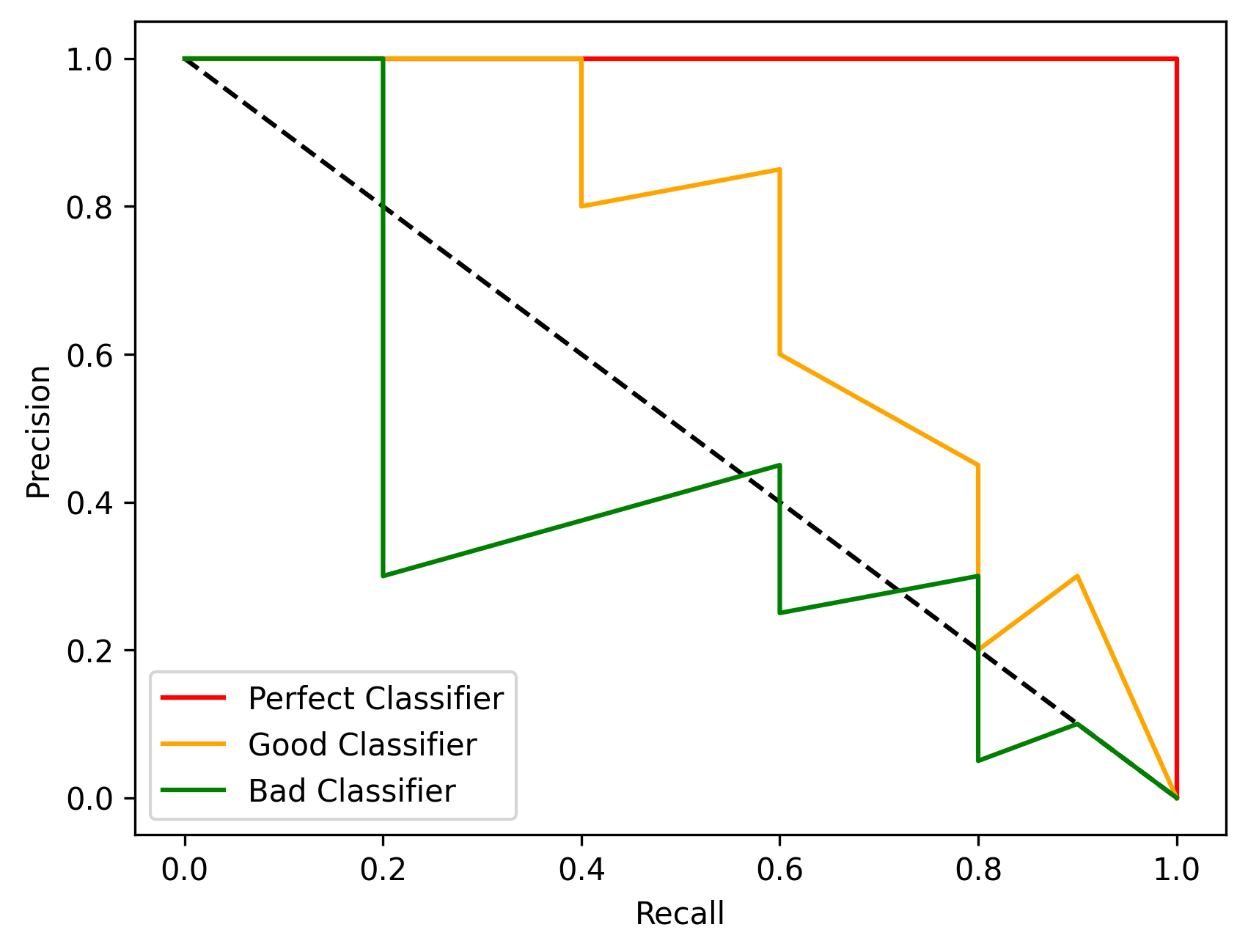# Precision-Recall Curve

The Precision-Recall curve evaluates the model performances at different threshold values by considering two parameters, the precision and the recall.### Example

``````from odin.classes import Curves

my_curve = Curves.PRECISION_RECALL_CURVE
# use my_curve as 'curve' parameter in the analyses
``````